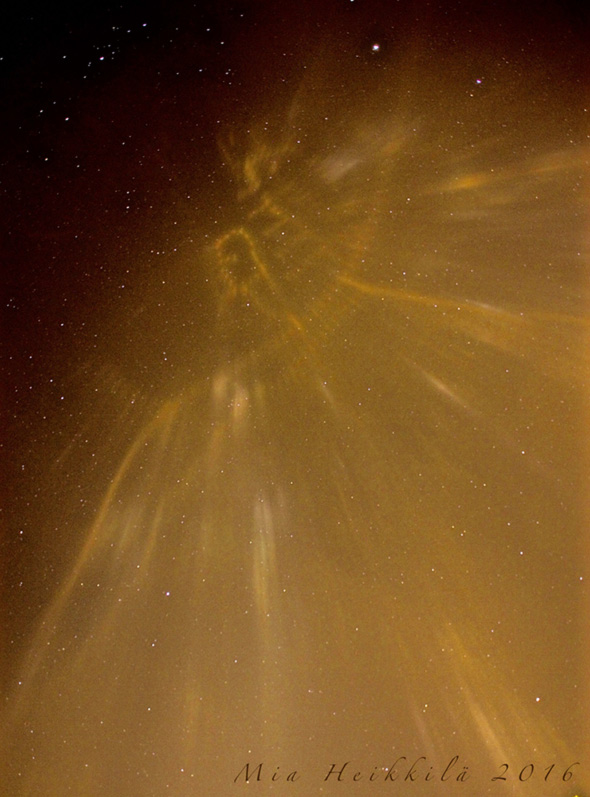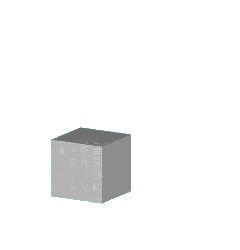## Diary - February 2016

#### February 1, 2016

You probably know about the sine and cosine. These are the most basic functions that are periodic: $$\sin(x + 2\pi) = \sin(x)$$ Elliptic functions are functions of two variables, $$x$$ and $$y$$, that are periodic in two directions. For example, we can have: $$f(x + 2\pi,y) = f(x,y)$$ and $$f(x,y + 2\pi) = f(x,y)$$ This movie is a way of illustrating an elliptic function.

What makes elliptic functions so special is that you can think of them as functions of a single complex variable: $$z = x + iy$$ and then they have a derivative in the special sense you learn about in a course on complex functions!

It's a lot harder for a complex function to have a derivative than an ordinary real function. A function like $$f(x,y) = \sin(x) \sin(y)$$ is periodic in two directions, but it doesn't have a derivative $$df/dz$$. Mysterious as this may sound, this is the reason elliptic functions are so special.

In the late 1800s, all the best mathematicians thought about elliptic functions, so there are 'Jacobi elliptic functions' and 'Weierstrass elliptic functions' and many more. Now they're less popular, but they're still incredibly important. You need to think about them if you want to deeply understand how long the perimeter of an ellipse is. They're also important in physics, and fundamental to the proof of Fermat's Last Theorem.

An elliptic function actually has a derivative everywhere except at certain points where the function 'blows up' — that is, becomes infinite. These points are called poles. You can prove an elliptic function has to have poles unless it is constant (and thus too boring to talk about).

Because an elliptic function is periodic in two directions, its poles make a repeating pattern in the plane, which you can see in this movie. The poles are the points from which checkerboard pattern keeps expanding outward. The zeros of the elliptic function — the points where it's zero — are the points where the checkerboard keeps shrinking inward.

For more on elliptic functions, you could try this:

The animation here was created by Gerard Westendorp.

#### February 4, 2016This is so cool I'm not sure I believe it. It's a photo of the night sky over a city in Finland. A rare atmospheric phenomenon called light pillars created a map of the city itself, in the sky!

Street lights were reflected back down by ice crystals in the air. This only happens when flat hexagonal crystals are floating horizontally in still air. Light bounces back down from the crystals.

This was taken on January 13, 2016, by Mia Heikkila in Eura, Finland. For more, read Phil Plait's article here:

He's a smart guy. If he believes this is real, I guess I do too.

You can compare this picture to a city map here:

#### February 18, 2016Inside every boring gray cube...

... there's a colorful dodecahedron yearning to unfold!

Puzzle. When we fold the dodecahedron back to a cube, does it fit together snugly, or is there some empty space left? What fraction of the cube is filled?

This animation was made by Hermann Serras, and I found it here:

I will give away the answer to the puzzle, because it's so pretty: there is empty space left, and the fraction of the cube that's filled is $$\Phi/2$$, where $$\Phi = \frac{\sqrt{5}+1}{2}$$ is the 'big' golden ratio. You can see some proofs in the comments to my Google+ post.

When you fold the dodecahedron into the cube, the shape of the empty space inside is called the concave pyrohedral dodecahedron or 'endo-docahedron'.

#### For my March 2016 diary, go here.

© 2016 John Baez
baez@math.removethis.ucr.andthis.edu